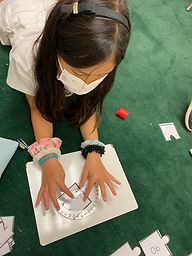## Ms. Kylie

### Target 1​

###### Lesson Type:

Continuation

Number Operation

:

Number Meaning

Understand that quantities can be either positive or negative.

###### 1:

Understand that positive and negative numbers occur in the real world.

###### 2:

Understand the relationship between positive and negative numbers.

###### 3:

Understand that integers get smaller in value as they move left in order, and larger as they move right in order.

6th

###### Vocabulary:

-Positive, Negative, Integer

Activities:

-Students were given an integer and identified where the integer would be on a number line.

-Students were given real world problems and identified if the amount was increasing (positive number) or decreasing (negative number).### Home Exploration

###### Guiding Questions:## Absent Students:

### Target 2

:

###### 1:

Accurately measure angles.

###### 2:

Identify angle types and estimate their measurement.

###### 3:

Understand that angles are measured in degrees (from 0 to 360).

4th

###### Vocabulary:

Angle, Degrees, Protractor

Activities:

-Students were given puzzle pieces that had angles and the degrees of angles. They measured the angles using a protractor and found the matching puzzle piece with the degree of the angle.

-Students were given the degrees of angles and practiced using the protractor to measure and draw the angle.### Home Exploration

###### Guiding Questions:### Target 3

:

###### Vocabulary:

Activities:### Home Exploration